## 5.18GAMG method

GAMG is an eﬀective form of multi-grid method used in ﬁnite volume CFD. It combines:

• geometric (“G”) agglomeration to deﬁne the structure of the coarse meshes;
• the algebraic multi-grid (AMG) method, where the matrix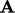is constructed at a coarser level from coeﬃcients at the ﬁner level, rather than by geometric data from the coarse mesh.

### Agglomeration

Pairwise agglomeration forms coarser meshes by joining pairs of cells at each level of coarsening. In a sweep through the cells, an unpaired cell is paired with the (unpaired) neighbour that shares the face with largest area. This method generally maintains a low aspect ratio (see Sec. 8.2 ) in the resulting agglomerated cells.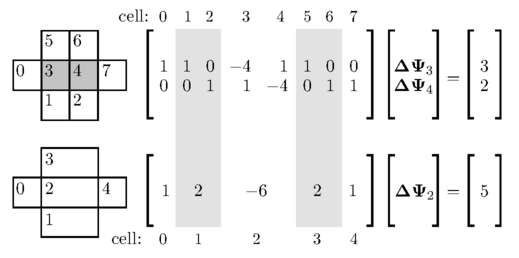The example shows an agglomeration of cell pairs 1-2, 3-4 and 5-6, which form cells 1, 2 and 3 respectively in the coarse mesh. If we solve the equation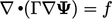on a 2D mesh of square cells with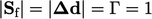, then Eq. (3.2 ) and Eq. (3.5 ) calculate diagonal coeﬃcients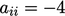and non-zero oﬀ-diagonal coeﬃcients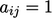.

Algebraic multi-grid creates coeﬃcients in the coarser mesh by summing coeﬃcientsand source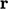from the ﬁner mesh. The example produces one row of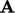which is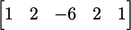.

If the Laplacian for the coarse mesh were discretised directly, the coeﬃcients in that row would be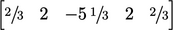due to increasing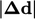. This discrepancy between agglomerated and calculated coeﬃcients is repeated in subsequent agglomerations.

Multi-grid begins solving/smoothing for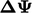(with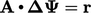) at the coarsest mesh. Coarse cell values ofare then injected into corresponding cells in the next ﬁnest mesh.

With a Laplacian term, the coeﬃcient discrepancy caused by algebraic agglomeration causesto be under-predicted. A correction tocan be applied by scaling it by a factor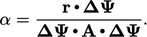(5.37)
This scaling is used when the equation being solved is dominated by a Laplacian term, e.g. a pressure equation, when summing coeﬃcients between agglomerated cells is inaccurate. Scaling is not generally used for transport dominated by advection, since there is no error associated with summing coeﬃcients from the advective derivative.

Equation 5.37 is derived by minimising the error with respect to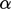in the equation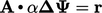. The minimisation is performed by setting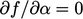for the quadratic form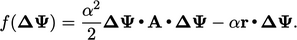(5.38)
Notes on CFD: General Principles - 5.18 GAMG method